Скачать презентацию 7 1 Exploring Exponential Models Learning goals

21b1263d8b54fb621900e3cbb87f7079.ppt

• Количество слайдов: 12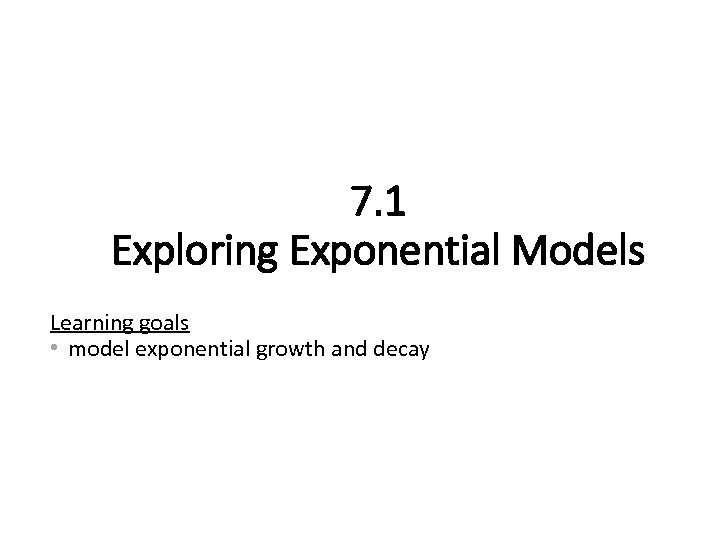7. 1 Exploring Exponential Models Learning goals • model exponential growth and decay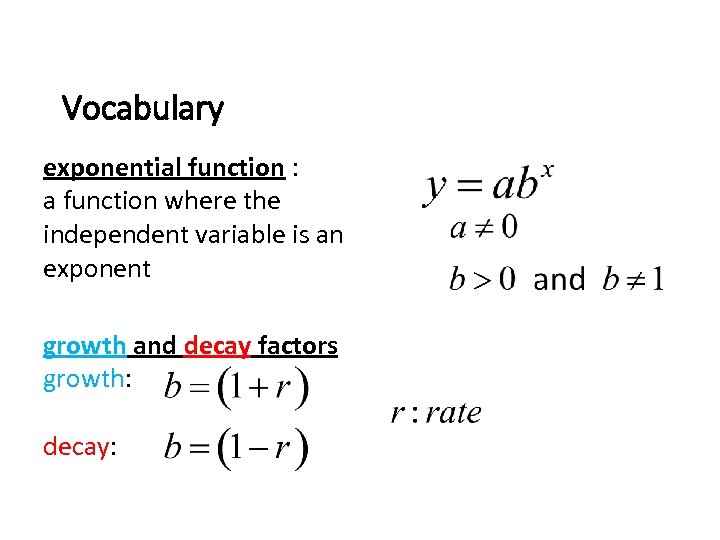Vocabulary exponential function : a function where the independent variable is an exponent growth and decay factors growth: decay: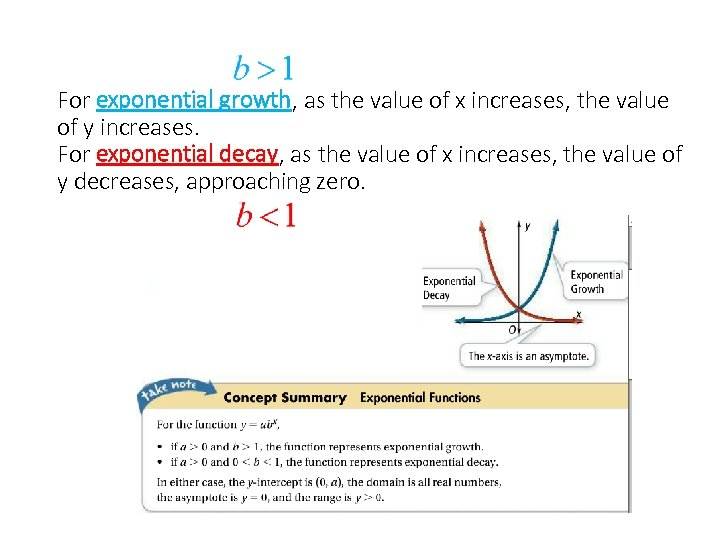For exponential growth, as the value of x increases, the value of y increases. For exponential decay, as the value of x increases, the value of y decreases, approaching zero.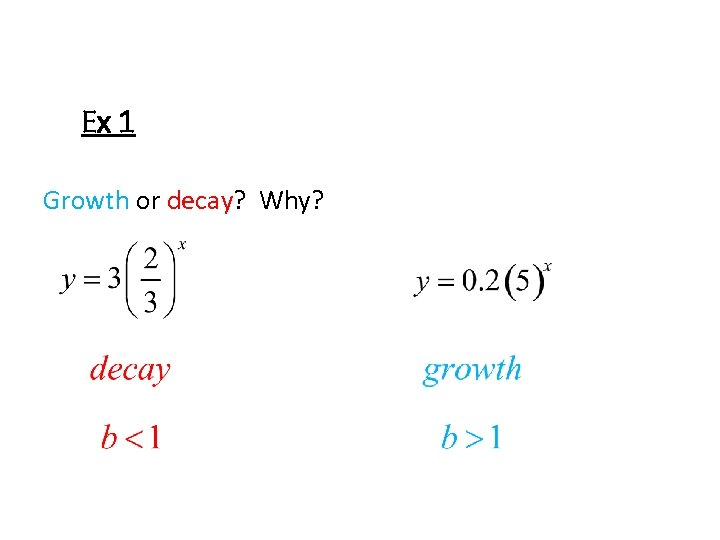Ex 1 Growth or decay? Why?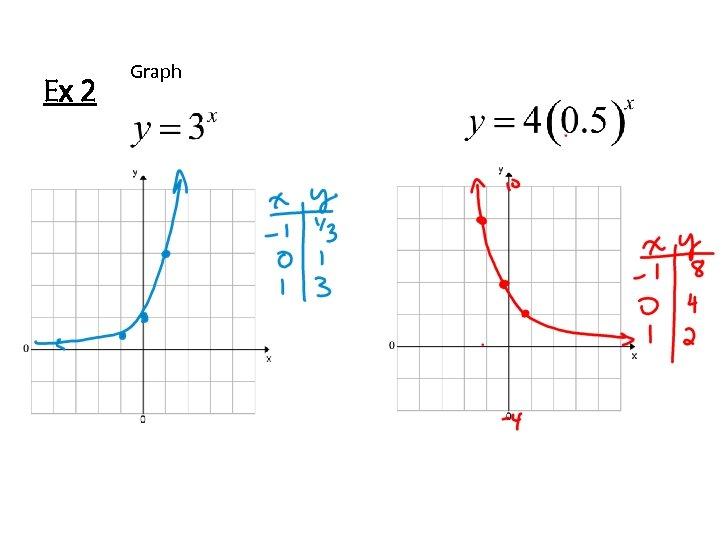Ex 2 Graph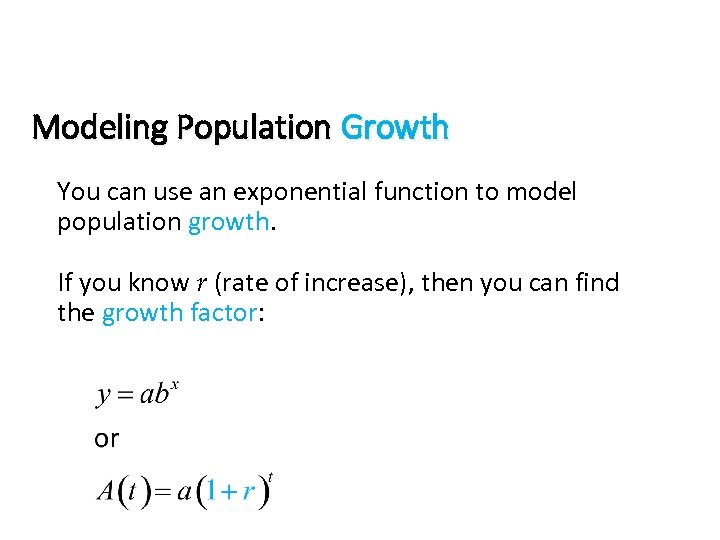Modeling Population Growth You can use an exponential function to model population growth. If you know r (rate of increase), then you can find the growth factor: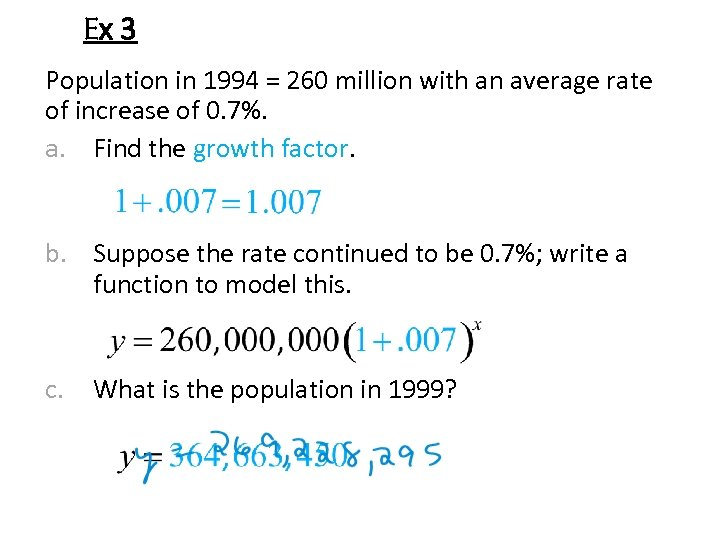Ex 3 Population in 1994 = 260 million with an average rate of increase of 0. 7%. a. Find the growth factor. b. Suppose the rate continued to be 0. 7%; write a function to model this. c. What is the population in 1999?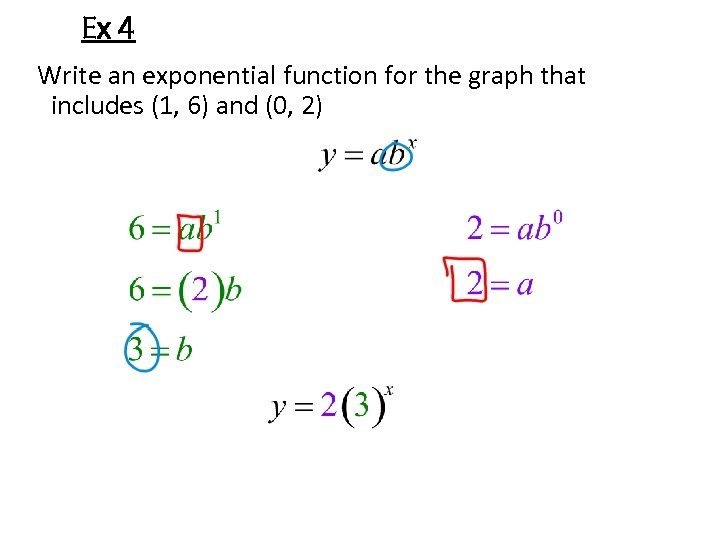Ex 4 Write an exponential function for the graph that includes (1, 6) and (0, 2)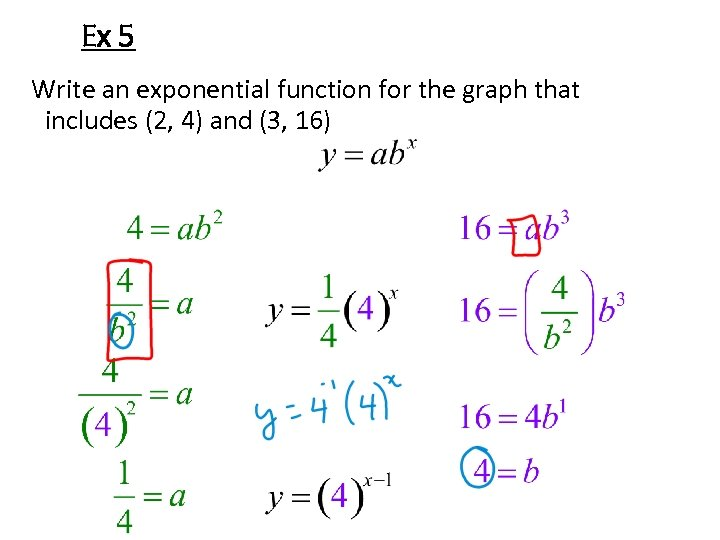Ex 5 Write an exponential function for the graph that includes (2, 4) and (3, 16)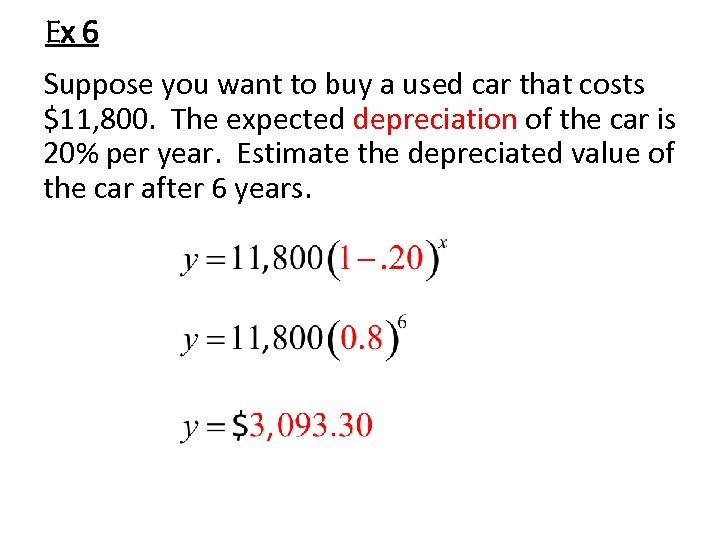Ex 6 Suppose you want to buy a used car that costs \$11, 800. The expected depreciation of the car is 20% per year. Estimate the depreciated value of the car after 6 years.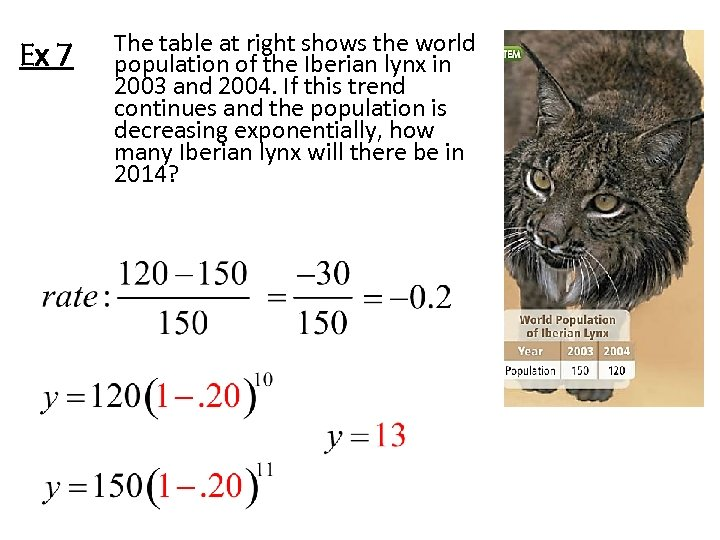Ex 7 The table at right shows the world population of the Iberian lynx in 2003 and 2004. If this trend continues and the population is decreasing exponentially, how many Iberian lynx will there be in 2014?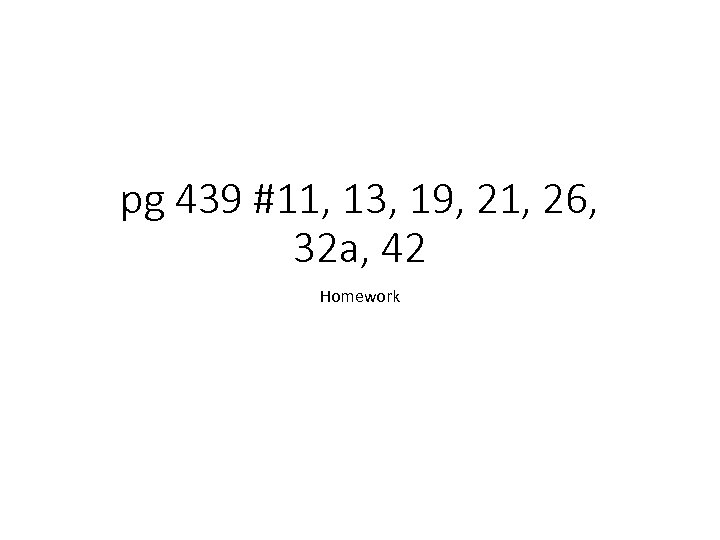pg 439 #11, 13, 19, 21, 26, 32 a, 42 Homework